Recently I began a project with the local primary cluster in developing materials for the new Curriculum for Wales. The first workbook was designed to meet the requirements of the algebra ‘what matters’ statement for progression step 2 (age 8).

In searching for tasks to include in the workbook, I wanted to include a purposeful practice exercise for recalling multiplication facts. Naturally, I had a look on the late Don Steward’s median blog, and came across the following task entitled “timestable”.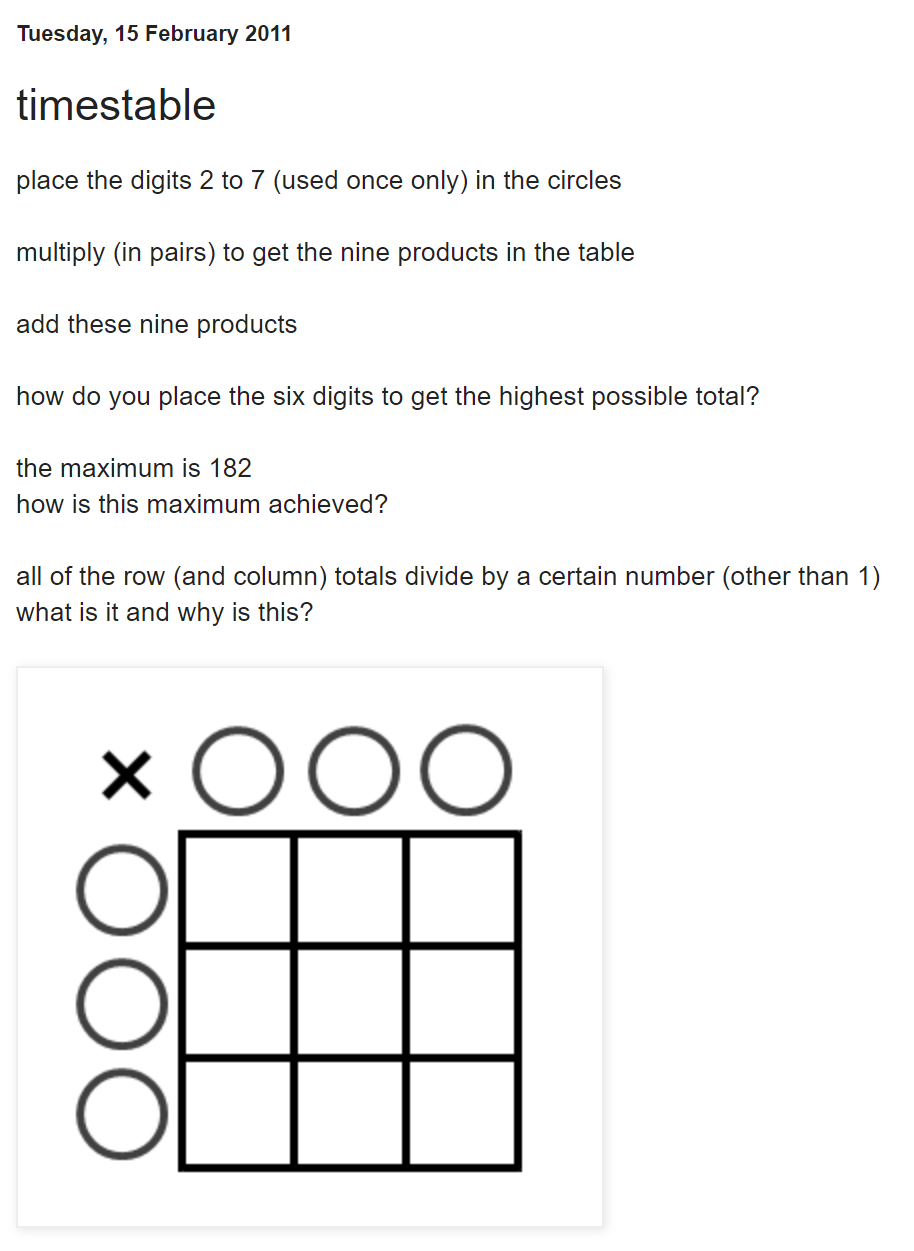Source: https://donsteward.blogspot.com/2011/02/timestable.html

The task was evidently low threshold, high ceiling, with some intriguing questions contained in the description.

Because progression step 2 only requires the knowledge of multiplication tables 2, 3, 4, 5 and 10, I needed to tweak the task to eliminate the need to multiply by 7. So, I changed the requirement to place the digits 2 to 7 in the circles to place the digits 1 to 6 in the circles.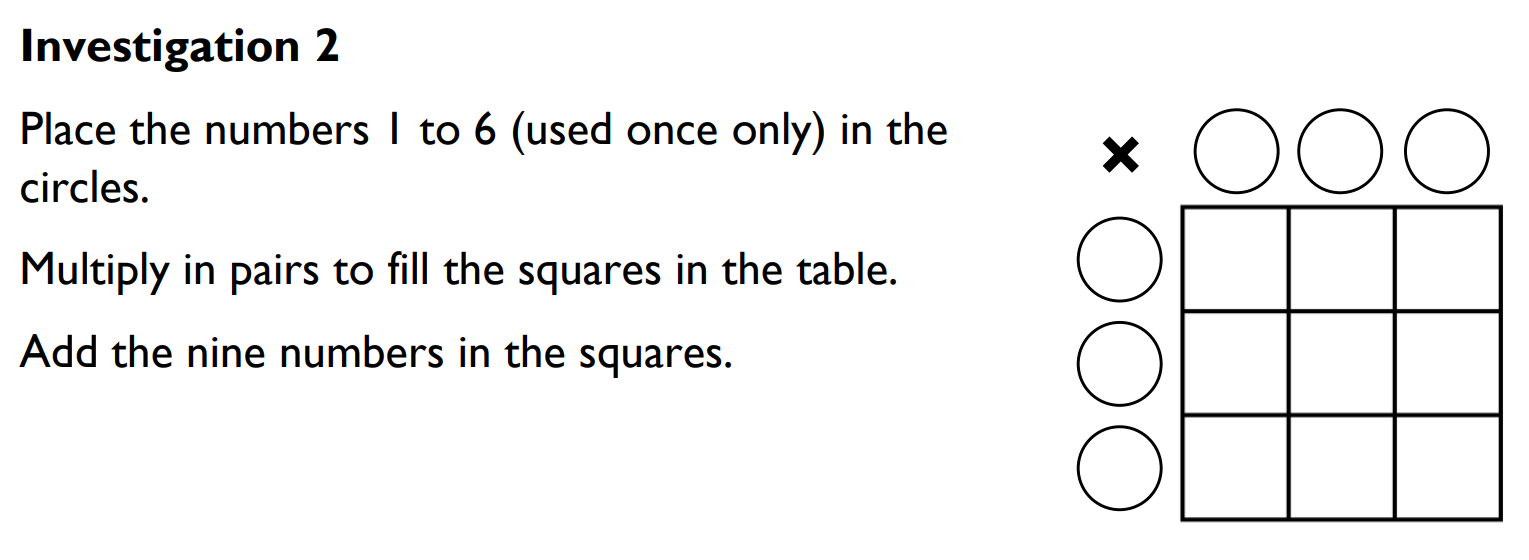I now needed to find the maximum possible total to match the original instructions. How to do this? You may like to think about this yourself before reading on…

I started by considering how many different grids can be formed. Assuming we start placing numbers in the bottom left and work clockwise, there are six choices for the number in the bottom left, 5 choices for the circle above, then 4 choices, 3 choices, 2 choices and finally a single choice. So, the total number of grids is

$$6 \times 5 \times 4 \times 3 \times 2 \times 1 = 720.$$

This can be written in mathematical notation as

$$6! = 720.$$

I did not fancy having to write out 720 grids! Even with the help of an Excel spreadsheet, this would be an arduous task…

Thinking of the general case, I used the variables $$a$$ to $$f$$ to represent the numbers placed in the circles.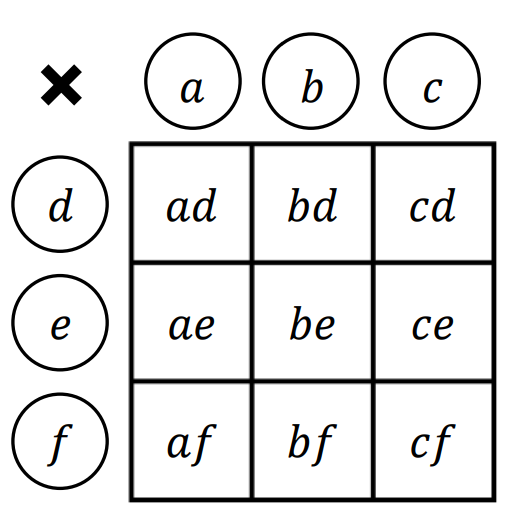The total of the nine numbers in the grid is

$$ad + bd + cd + ae + be + ce + af + bf + cf$$

$$=d(a + b + c) + e(a + b + c) + f(a + b + c)$$

$$=(a + b + c)(d + e + f).$$

This allows us to narrow down the number of grids to consider, as it does not matter how the columns are ordered, or how the rows are ordered (the total $$a + b + c$$ will be the same regardless of how we order the numbers $$a$$, $$b$$ and $$c$$ in the columns).

There are $$3 \times 2 \times 1 = 6$$ ways to order three numbers, so the number of grids we need to consider goes down from 720 to

$$\frac{6!}{3! \times 3!} = 20.$$

This is within the realms of possibility!

Assuming that we always write $$a$$, $$b$$, $$c$$ in order from least to greatest, and also $$d$$, $$e$$, $$f$$ in order from least to greatest, there are 10 possible grids in which $$a=1$$: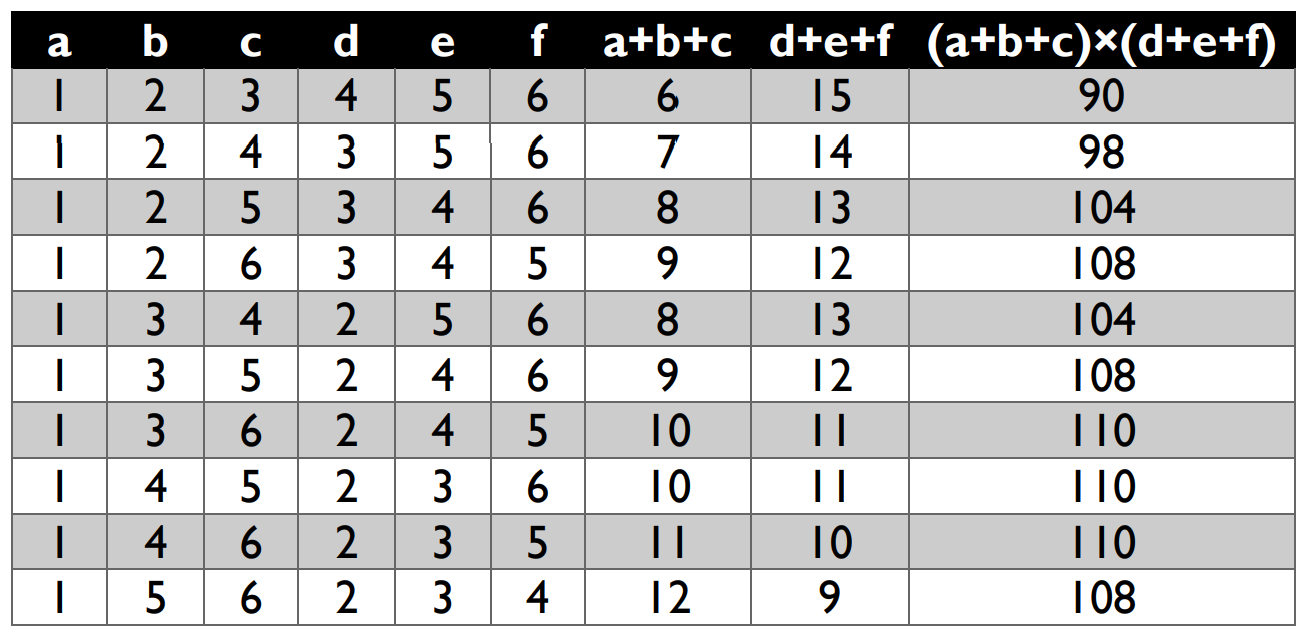It turns out that there are no other grids, because if the number 1 appears as $$d$$, $$e$$ or $$f$$, by symmetry (swapping rows to columns) we have already considered it in the table above. So, from an initial 720 grids, we have narrowed it down to 10 unique grids. The final column shows us that there are 5 unique totals, namely 90; 98; 104; 108 and 110. Isn’t that neat! The wording for the final task reflects this fact: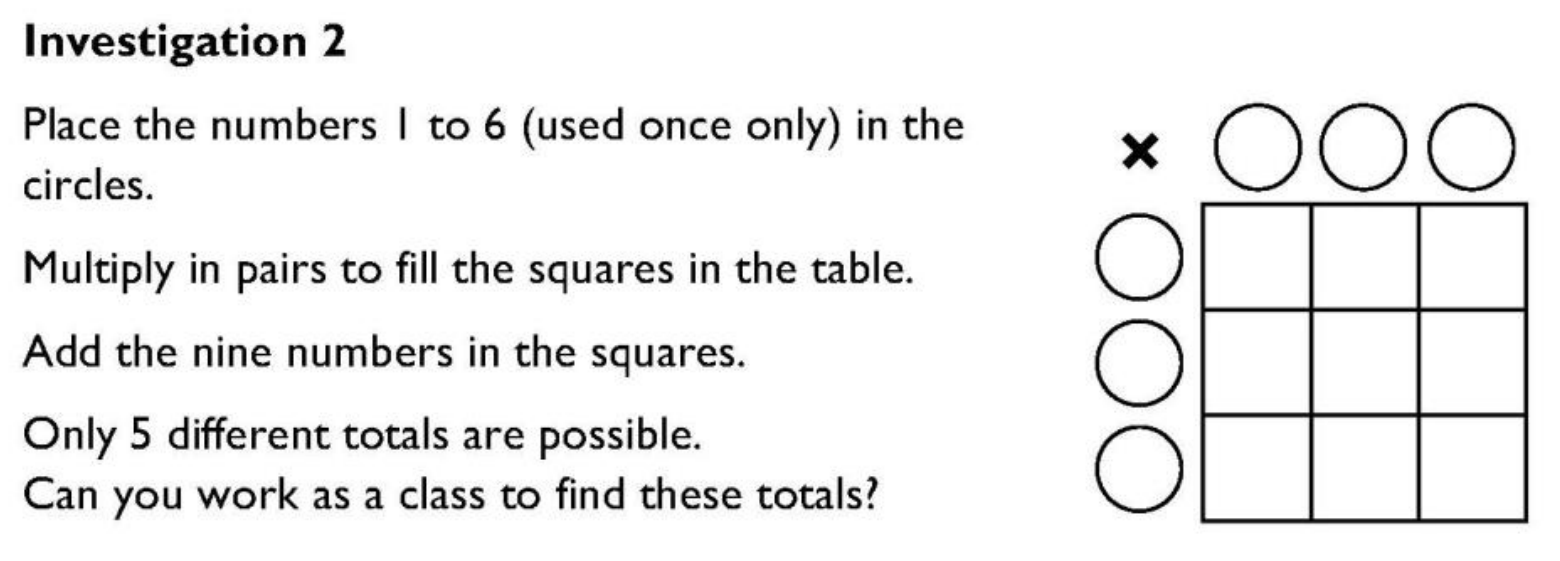Recently I was teaching proof to Year 12 and used this task to demonstrate proof by exhaustion ("prove that this task has exactly 5 different totals"). Isn’t it lovely that the same task can be used all the way from Year 3 to Year 12?

Diweddarwyd ddiwethaf: Gwener, 9 Ebrill 2021, 10:33 am